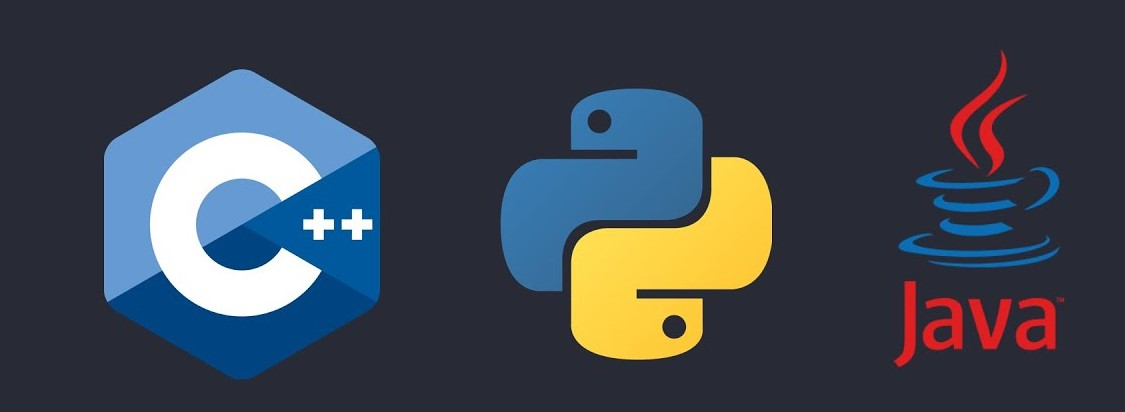# Comparing Python, C++, and Java - Unraveling the Differences

## Introduction

The programming language landscape is rich and diverse, offering a plethora of options to suit various needs. Python, C++, and Java have emerged as prominent languages, each with distinct attributes catering to diverse programming scenarios. This blog provides an in-depth comparison of Python, C++, and Java, delving into their syntax, features, and providing code examples to highlight their differences.

Python is celebrated for its readability and versatility. Its concise syntax and dynamic typing make it a favorite for a wide range of applications.

``````# Python Example: Calculating the Fibonacci Sequence
def fibonacci(n):
if n <= 0:
return []
elif n == 1:
return 
elif n == 2:
return [0, 1]
else:
fib_sequence = [0, 1]
while len(fib_sequence) < n:
next_num = fib_sequence[-1] + fib_sequence[-2]
fib_sequence.append(next_num)
return fib_sequence

print(fibonacci(10))
``````

## C++: Power and Flexibility

C++ combines performance with flexibility. It offers low-level control and high-level features, making it suitable for systems programming and resource-intensive applications.

``````// C++ Example: Calculating the Fibonacci Sequence
#include <iostream>
using namespace std;

int main() {
int n = 10;
int fib_sequence[n];

fib_sequence = 0;
fib_sequence = 1;

for (int i = 2; i < n; i++) {
fib_sequence[i] = fib_sequence[i - 1] + fib_sequence[i - 2];
}

for (int i = 0; i < n; i++) {
cout << fib_sequence[i] << " ";
}

return 0;
}
``````

## Java: Object-Oriented and Platform-Independent

Java's strength lies in its object-oriented design and platform independence achieved through the Java Virtual Machine (JVM).

``````// Java Example: Calculating the Fibonacci Sequence
public class Fibonacci {
public static void main(String[] args) {
int n = 10;
int[] fibSequence = new int[n];

fibSequence = 0;
fibSequence = 1;

for (int i = 2; i < n; i++) {
fibSequence[i] = fibSequence[i - 1] + fibSequence[i - 2];
}

for (int num : fibSequence) {
System.out.print(num + " ");
}
}
}
``````

## Comparing Features

FeaturePythonC++Java
TypingDynamicStaticStatic
Memory ControlAutomaticManualAutomatic
Object-OrientedYesYesYes
Platform-IndependenceNoNoYes
SyntaxConciseIntermediateIntermediate
CommunityStrongRobustRobust
PerformanceModerateHighModerate

## Choosing the Right Language

• Python: For rapid development, data analysis, scripting, and applications where readability is crucial.

• C++: For resource-intensive applications, systems programming, and projects demanding a balance of performance and features.

• Java: For building platform-independent applications, web development, and projects that require strong object-oriented design.

## Conclusion

Python, C++, and Java exhibit their unique strengths, addressing a diverse range of programming requirements. Whether it's the readability of Python, the power of C++, or the platform independence of Java, each language offers distinct advantages. The choice among these languages hinges on project needs, programming preferences, and the specific domain. By comprehending their characteristics, you can make an informed decision aligned with your coding endeavors.Rohit Kushwaha

# [email protected]

Web DeveloperFreelancer